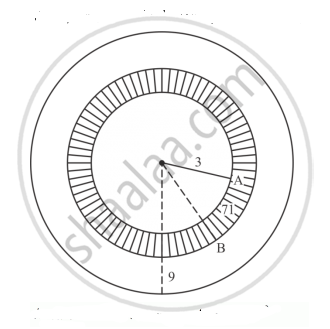Share

# A Target Shown in Fig. Below Consists of Three Concentric Circles of Radii, 3, 7 and 9 Cm Respectively. a Dart is Thrown and Lands on the Target. What is the Probability that the Dart Will Land on the Shaded Region? - CBSE Class 10 - Mathematics

ConceptProbability Examples and Solutions

#### Question

A target shown in Fig. below consists of three concentric circles of radii, 3, 7 and 9 cm
respectively. A dart is thrown and lands on the target. What is the probability that the dart will land on the shaded region?#### Solution

1st circle ⟶ with radius 3
2nd circle ⟶ with radius 7
3rd circle ⟶ with radius 9
Area of 1st circle = (3)^2 = 9𝜋
Area of 2nd circle= (7)^2 = 49𝜋
Area of 3rd circle = (9)^2 = 81𝜋
Area of shaded region = Area of 2nd circle – area of 1st circle
= 49𝜋 – 9𝜋
= 40𝜋
Probability that will land on the shaded region ="area of shaded region"/"area of 3rd circle"= (40pi) /(81pi) = 40/81

Is there an error in this question or solution?

#### Video TutorialsVIEW ALL 

Solution A Target Shown in Fig. Below Consists of Three Concentric Circles of Radii, 3, 7 and 9 Cm Respectively. a Dart is Thrown and Lands on the Target. What is the Probability that the Dart Will Land on the Shaded Region? Concept: Probability Examples and Solutions.
S